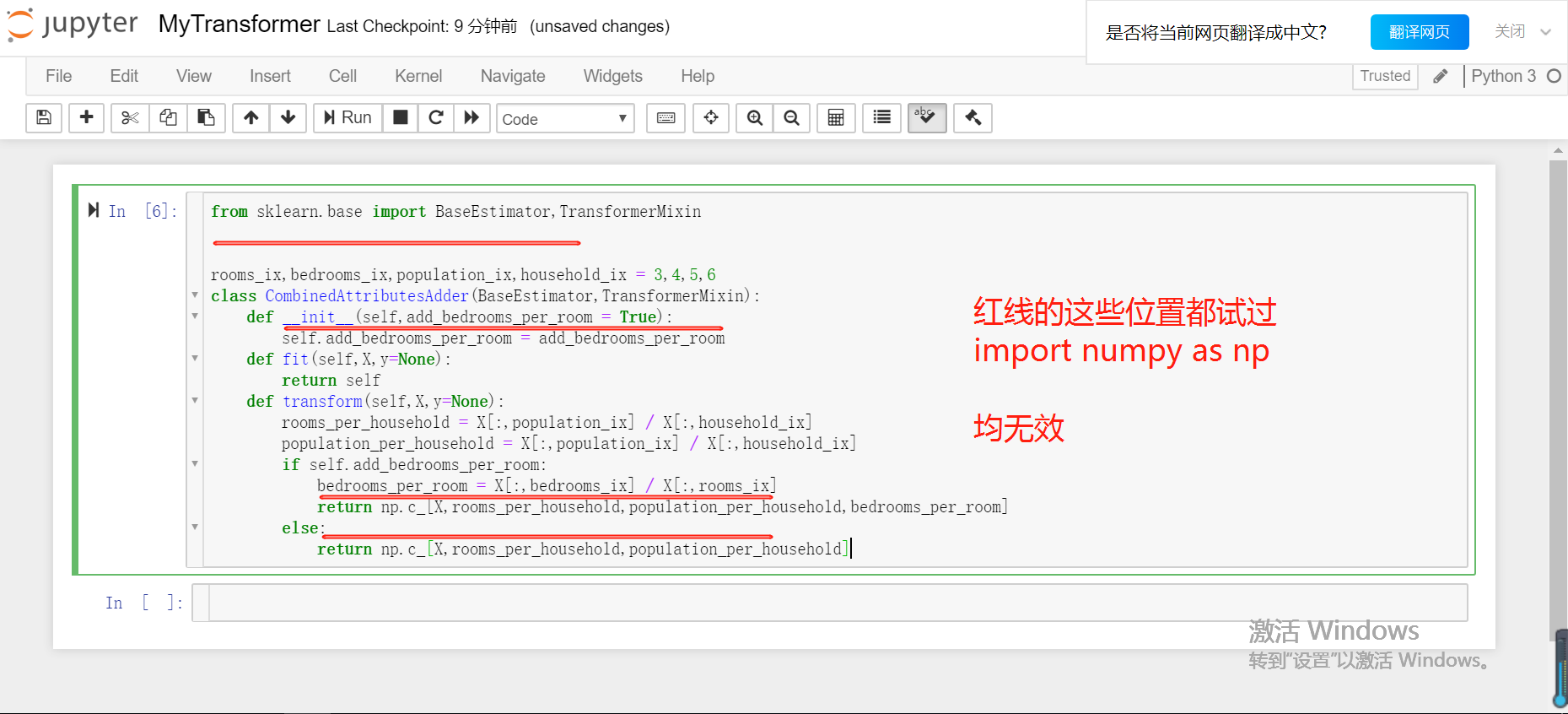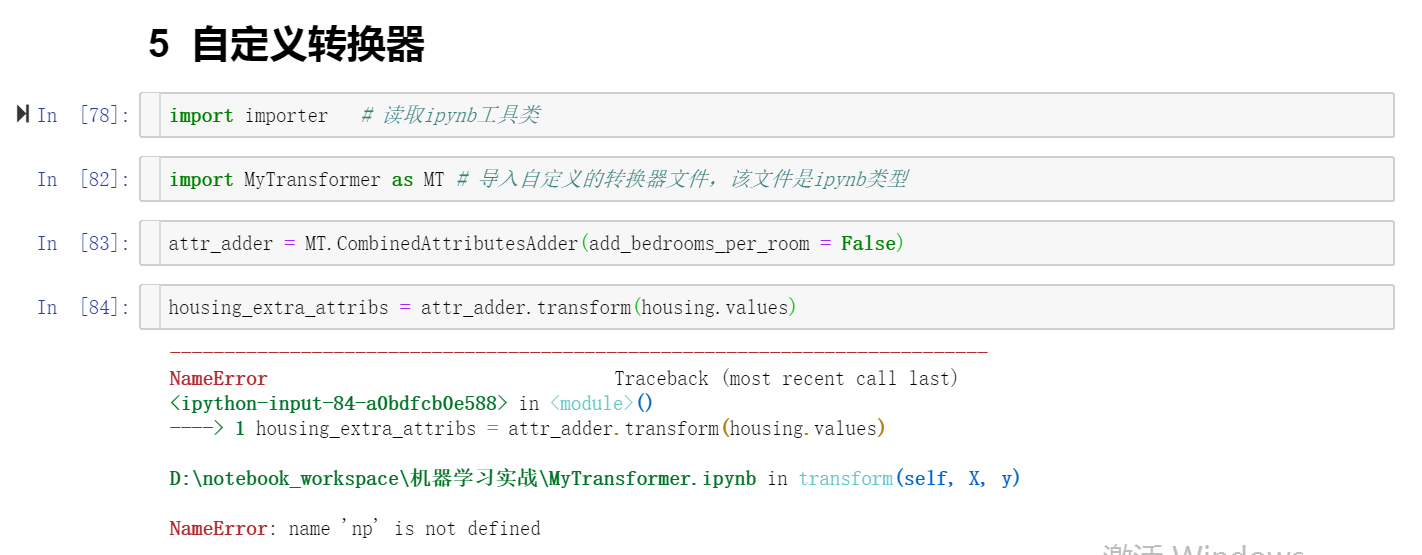2个回答

import numpy as npgreatofdream 回复qq_38018783: 它这个问题是需要重新加载库的原因，我的回答不完整
8 个月之前 回复qq_38018783 谢谢，已解决~
8 个月之前 回复10 个月之前 回复greatofdream 回复苝花向暖丨楠枝向寒: 你导入后shift+enter执行了吗？
10 个月之前 回复10 个月之前 回复python方法中调用函数出错 NameError: name 'get_step' is not defined
NameError: name 'lsx' is not defined
lsx,lsy在函数内定义： ``` def date(): lsx=[] dates=file.split("\n") for date in dates: if "-" in date: if date.replace("-","").isnumeric()==True: p1=date.index('-')#the first - p2=date.find('-',p1+1)#the second - month=date[p1+1:p2] day=date[p2+1:] date_on_x=float(month+"."+day) lsx.append(date_on_x-50) def coloring(): lsy=[] lines=file.split("重庆") i=0 for line in lines: #index the temprature inn=line.index('\n')#The first \n inc=line.index("C")#The first C if i==0: tu=int(line[line.find('\n',inn+1)+1:inc])#The second \n if "～" in line: tl=int(line[line.index('～')+1:line.rindex('C')]) else: tl=tu i=i+1 else: fn=line.find('\n',inn+1) tu=int(line[line.find('\n',fn+1)+1:inc])#The third \n if "～" in line: tl=int(line[line.index('～')+1:line.rindex('C')]) else: tl=tu t=(tl+tu)/2#daily average temprature if t<8: turtle.color("purple") elif 8<=t<12: turtle.color("lightblue") elif 12<=t<22: turtle.color("green") elif 22<=t<28: turtle.color("yellow") elif 28<=t<30: turtle.color("orange") elif t>=30: turtle.color("red") lsy.append(t) ``` 在另一个函数那里调用： ``` def rainbow(): global lsx,lsy #let's draw! for i in zip(lsx,lsy): turtle.pu() turtle.goto(i) turtle.pd() coloring() turtle.circle(10) ``` 报错为： ``` Traceback (most recent call last): File "C:\Users\jyz_1\AppData\Local\Programs\Python\Python37-32\32rx.py", line 105, in <module> rainbow() File "C:\Users\jyz_1\AppData\Local\Programs\Python\Python37-32\32rx.py", line 93, in rainbow for i in zip(lsx,lsy): NameError: name 'lsx' is not defined ``` 请求解决方案
NameError: name 'v_xs' is not defined
NameError: name 'v_xs' is not defined y_pre = sess.run(prediction,feed_dict={xs:v_xs}) 在spyder下可以运行，ubuntu终端 链接服务器 下运行出现以上错误 请问如何解决？
python的NameError: name 'xx‘ is not defined该怎么解决？

NameError: name 'clf' is not defined”
import sys,random,os,pickle from multiprocessing import Pool from sklearn.svm import SVC from sklearn import metrics from sklearn import cross_validation from sklearn.cross_validation import train_test_split from sklearn.externals import joblib import pandas as pd train = pd.read_csv("E:/training38085.csv") test = pd.read_csv("E:/test38085.csv") test_x = test.drop("bad_good",axis=1) #生成validation set val = pd.read_csv('E:/validation20000_950.csv') train_y = train.bad_good train_x = train.drop(['bad_good'],axis=1) val_y = val.bad_good val_x = val.drop(['bad_good'],axis=1) print(test_x.shape) print(train_x.shape) print(val_x.shape) def pipeline(iteration,C,gamma,random_seed): x_train, _x , y_train, _y = train_test_split(train_x,train_y,test_size=0.4,random_state=random_seed) print(x_train.shape) clf = SVC(C=C,kernel='rbf',gamma=gamma,probability=True,cache_size=7000,class_weight='balanced',verbose=True,random_state=random_seed) clf.fit(x_train,y_train) #predict test set pred = clf.predict_proba(test_x) Traceback (most recent call last): File "<pyshell#22>", line 1, in <module> 报错： “pred = clf.predict_proba(test_x) NameError: name 'clf' is not defined” 上面def pipline 有clf ,为啥还会报错呢。前面的代码没有报错
pytorch 中 写入class ConvNet(nn.Module): 语句之后 出现错误NameError: name 'ConvNet' is not defined 这是怎么回事？
python使用scapy，使用发包工具时，报错NameError: name 'udp' is not defined，求解答，
``` #!env python # -*- coding: <encoding name> -*- import sys from scapy.all import * import os,random,datetime,time,math from random import randrange from random import uniform from functools import reduce def create_data(line): current_time = [ datetime.datetime.now().strftime('%b %d %H:%M:%S'), datetime.datetime.now().strftime('%Y-%m-%d %H:%M:%S'), # datetime.datetime.now().strftime('%b %d %H:%M:%S %Y'), str(math.trunc(datetime.datetime.now().timestamp())) ] regular = [ "(\w{3}\s+\d{1,2}\s+\d{1,2}:\d{1,2}:\d{1,2})", "(\d{4}-\d{1,2}-\d{1,2}\s+\d{1,2}:\d{1,2}:\d{1,2})", "(\w{3}\s+\d{1,2}\s+\d{1,2}:\d{1,2}:\d{1,2}\s+\d{1,4})", "(\d{10})" ] def current_time(line): for i in regular: for j in current_time(line): if len(re.findall(i, j)) != 0: #若正则匹配上相对应的时间格式，则对字符串进行替换 line=re.sub(i,j,line) return line dict_ip_logfile={ "192.168.58.84": r"C:\Users\CS\Desktop\HPHIDS.txt" } while True: for ip,logfile in dict_ip_logfile.items(): with open(logfile,"r",encoding='unicode_escape') as log: for line in log: print(ip) print(logfile) print(create_data(line)) # IP = (porto='udp','192.168.57.45') # UDP = (dst='192.168.57.14',8089) # scapy.all,send(IP,udp) scapy.all.send(IP(proto="udp",src=ip,dst="192.168.57.45")/udp(dst='192.168.57.14.',dport=8082)/line,inter=5,loop=1,count=2) log.close() ``` ![图片说明](https://img-ask.csdn.net/upload/201911/21/1574303068_105938.png)

import matplotlib.pyplot as plt from sklearn.model_selection import train_test_split from numpy import hstack from numpy import zeros from numpy import ones from numpy.random import rand from numpy.random import randn from keras.models import Sequential from keras.layers import Dense def define_discriminator(n_inputs=2): model=Sequential() model.add(Dense(25, activation='relu',kernel_initializer='he_uniform',input_dim=n_inputs)) model.add(Dense(1,activation='sigmoid')) model.compile(loss='binary_crossentropy',optimizer='adam', metrics=['accuracy']) return model def define_generator(latent_dim,n_outputs=2): model=Sequential() model.add(Dense(15, activation='relu',kernel_initializer='he_uniform', input_dim=latent_dim)) model.add(Dense(n_outputs,activation='linear')) return model def define_gan(generator,discriminator): discriminator.trainable=False model=Sequential() model.add(generator) model.add(discriminator) model.compile(loss='binary_crossentropy',optimizer='adam') return model def generate_real_samples(n): x1=rand(n)-0.5 x2=x1*x1 x1=x1.reshape(n,1) x2=x2.reshape(n,1) x=hstack((x1,x2)) y=ones((n,1)) return x,y def generate_latent_points(latent_dim,n): x_input=randn(latent_dim*n) x_input=x_input.reshape(n,latent_dim) return x_input def generate_fake_samples(generator,latent_dim,n): x_input=generate_latent_points(latent_dim,n) x=generator.predict(x_input) y=zeros((n,1)) return x,y def summarize_performance(epoch,generator,discriminator,latent_dim,n=100): x_real,y_real=generate_real_samples(n) _,acc_real=discriminator.evaluate(x_real,y_real,verbose=0) x_fake, y_fake = generate_fake_samples(generator,latent_dim,n) _, acc_fake = discriminator.evaluate(x_fake, y_fake, verbose=0) print(epoch,acc_real,acc_fake) plt.scatter(x_real[:,0],x_real[:,1],color='red') plt.scatter(x_fake[:, 0], x_fake[:, 1], color='blue') plt.show() def train(g_model,d_model,gan_model,latent_dim,n_epochs=10000,n_batch=128,n_eval=2000): half_batch=int(n_batch/2) for i in range(n_epochs): x_real,y_real=generate_real_samples(half_batch) x_fake,y_fake=generate_fake_samples(g_model,latent_dim,half_batch) d_model.train_on_batch(x_real,y_real) d_model.train_on_batch(x_fake, y_fake) x_gan=generate_latent_points(latent_dim,n_batch) y_gan=ones((n_batch,1)) gan_model.train_on_batch(x_gan,y_gan) if(i+1)%n_epochs==0: summarize_performance(i,g_model,d_model,latent_dim) latent_dim=5 discriminator=define_discriminator() generator=define_generator(latent_dim) gan_model=define_gan(generator,discriminator) train(generator,discriminator,gan_model,latent_dim) 问题
NameError: name 'label_train' is not defined

python报错问题nameerror
nameerror：name GrackGeetest is not defined 查看了程序没有任何的错误，单词也没有拼写错误，GrackGeetest名字就是类名，没有任何问题 还有其他解决问题吗？
python 中 我自定义了 两个函数 但是调用的时候说报错说未定义

tkinter程序运行时报错

python3中的_name_变量

python3.4 "_" eclipse 运行
python3.4 "_" eclipse 运行 NameError: name '_' is not defined 求过来人解答，为何
python赋值出出错，怎么解决新人求帮助
import urllib.request data=urllib.request.urlopen("http://edu.csdn.net").read() 执行date报错，怎么解决 Traceback (most recent call last): File "<pyshell#1>", line 1, in <module> date NameError: name 'date' is not defined
python 类对象中静态属性的作用域与模块中全局变量的作用域差别
1.之前我理解类是为了节省时间演化来的，因此里面的类的静态属性应该和模块中的全局变量作用范围类似，那类中的静态属性应该在整个类中都能访问。但实际为代码段所示。 2. ``` 2.1 全局变量能被内部定义函数所访问： c=10 def glass(x): return x*c >>> glass(5) #能访问全局变量 50 ``` ``` 2.实例对象中的方法直接访问静态属性： class A: c=10 def glass(self,x): return x*c #除非改成return x*self.c >>> a=A() >>> a.glass(5) Traceback (most recent call last): File "<pyshell#1>", line 1, in <module> a.glass(5) File "F:/new/new world/ex45/delete.all1.py", line 4, in glass return x*c NameError: name 'c' is not defined ``` 3. 疑惑点：为什么有这样的差别呢？难道在类对象中，只能通过self.的形式来传递属性或者方法吗？

get_ipython().run_line_magic('matplotlib', 'inline') NameError: name 'get_ipython' is not defined

Lintcode name 'Solution' is not defined EXITCODE=1

Java学习的正确打开方式

Spring Boot2 系列教程(十七)SpringBoot 整合 Swagger2

Python 基础（一）：入门必备知识

Python十大装B语法
Python 是一种代表简单思想的语言，其语法相对简单，很容易上手。不过，如果就此小视 Python 语法的精妙和深邃，那就大错特错了。本文精心筛选了最能展现 Python 语法之精妙的十个知识点，并附上详细的实例代码。如能在实战中融会贯通、灵活使用，必将使代码更为精炼、高效，同时也会极大提升代码B格，使之看上去更老练，读起来更优雅。 1. for - else 什么？不是 if 和 else 才

2019年11月中国大陆编程语言排行榜
2019年11月2日，我统计了某招聘网站，获得有效程序员招聘数据9万条。针对招聘信息，提取编程语言关键字，并统计如下： 编程语言比例 rank pl_ percentage 1 java 33.62% 2 c/c++ 16.42% 3 c_sharp 12.82% 4 javascript 12.31% 5 python 7.93% 6 go 7.25% 7

2020 网络课 智慧树自动刷课代码，自动跳转，自动答题并关闭弹窗，自动1.5倍速静音

SQL-小白最佳入门sql查询一

“狗屁不通文章生成器”登顶GitHub热榜，分分钟写出万字形式主义大作

IT界知名的程序员曾说：对于那些月薪三万以下，自称IT工程师的码农们，其实我们从来没有把他们归为我们IT工程师的队伍。他们虽然总是以IT工程师自居，但只是他们一厢情愿罢了。 此话一出，不知激起了多少(码农)程序员的愤怒，却又无可奈何，于是码农问程序员。 码农：你知道get和post请求到底有什么区别？ 程序员：你看这篇就知道了。 码农：你月薪三万了？ 程序员：嗯。 码农：你是怎么做到的? 程序员：

《程序人生》系列-这个程序员只用了20行代码就拿了冠军

8年经验面试官详解 Java 面试秘诀
作者 | 胡书敏 责编 | 刘静 出品 | CSDN（ID：CSDNnews） 本人目前在一家知名外企担任架构师，而且最近八年来，在多家外企和互联网公司担任Java技术面试官，前后累计面试了有两三百位候选人。在本文里，就将结合本人的面试经验，针对Java初学者、Java初级开发和Java开发，给出若干准备简历和准备面试的建议。   Java程序员准备和投递简历的实

1.两种思维方式在求职面试中，经常会考察这种问题：北京有多少量特斯拉汽车？ 某胡同口的煎饼摊一年能卖出多少个煎饼？ 深圳有多少个产品经理？ 一辆公交车里能装下多少个乒乓球？ 一